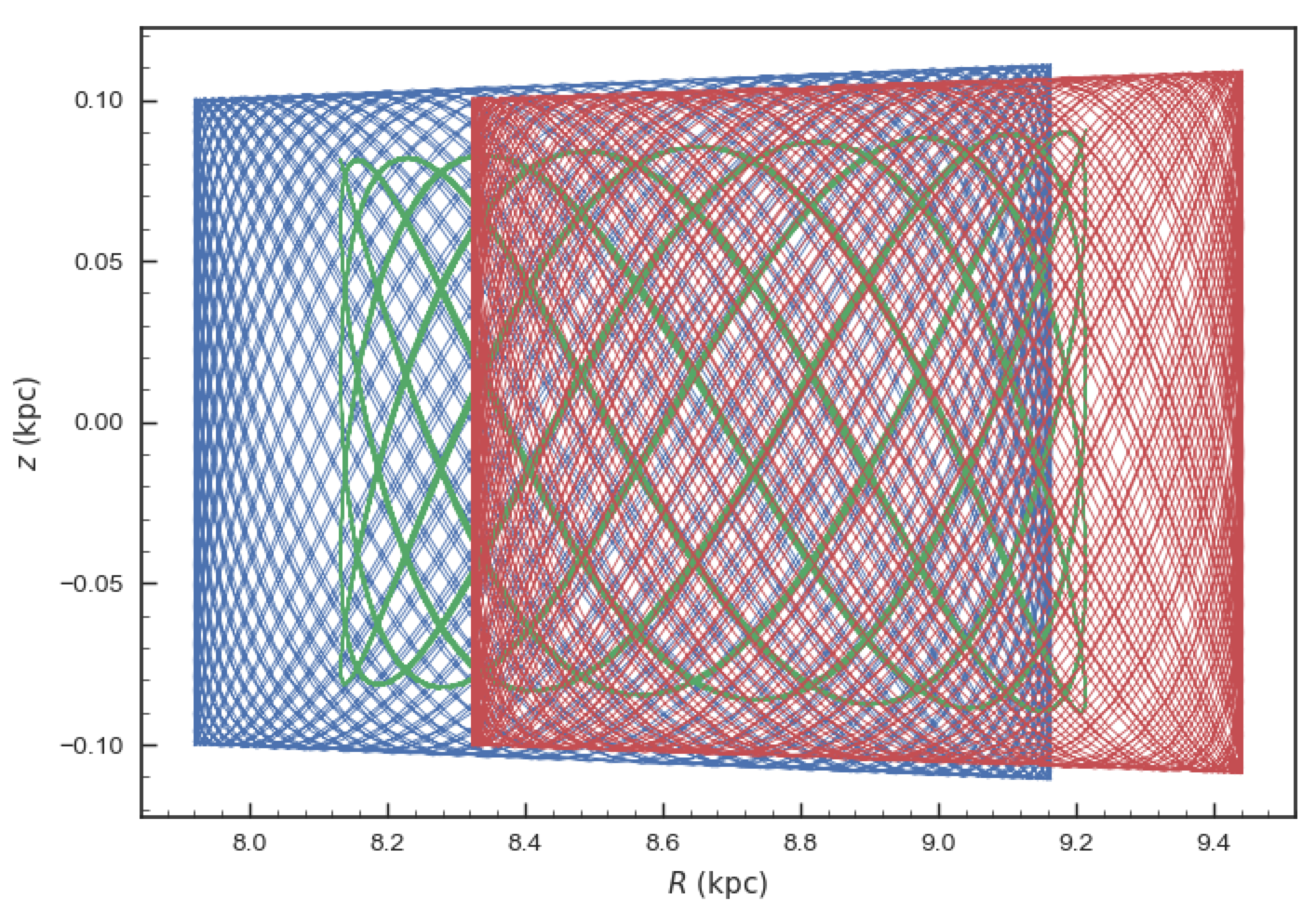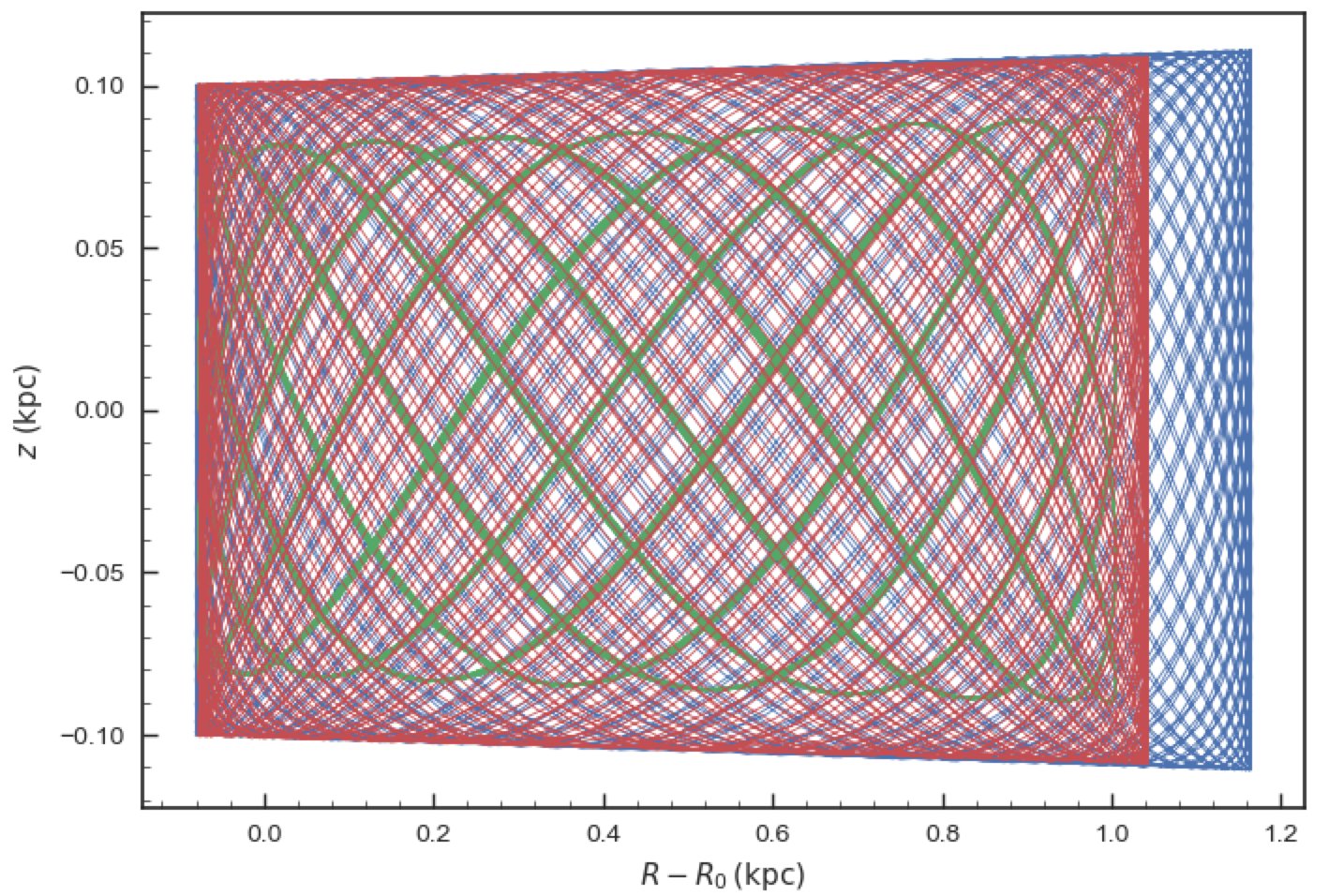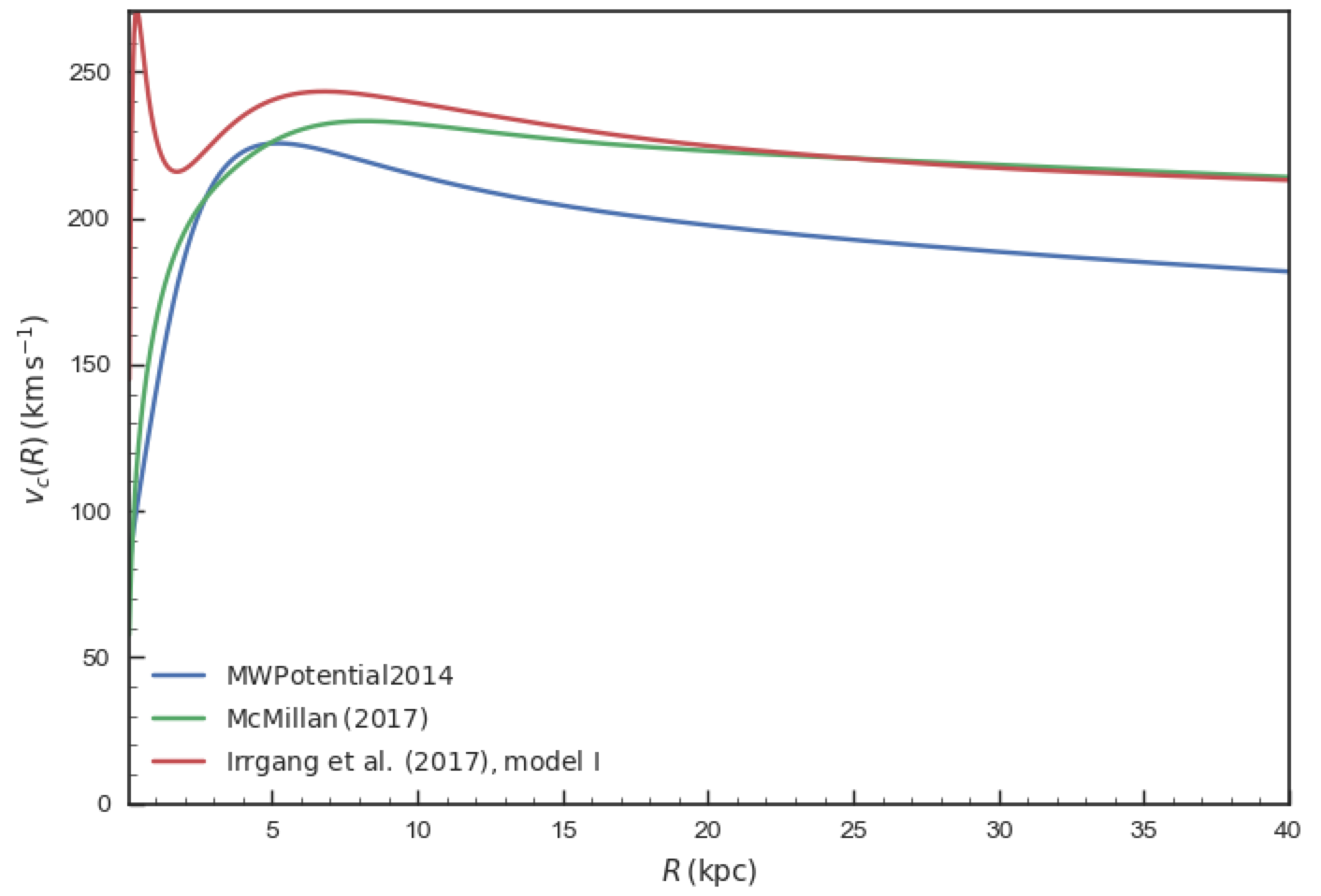# Potential (galpy.potential)¶

## 3D potentials¶

### General instance routines¶

Use as Potential-instance.method(...)

In addition to these, the NFWPotential also has methods to calculate virial quantities

### General 3D potential routines¶

Use as method(...)

In addition to these, the following methods are available to compute expansion coefficients for the SCFPotential class for a given density

### Specific potentials¶

All of the following potentials can also be modified by the specific WrapperPotentials listed below.

#### Ellipsoidal triaxial potentials¶

galpy has very general support for implementing triaxial (or the oblate and prolate special cases) of ellipsoidal potentials through the general EllipsoidalPotential class. These potentials have densities that are uniform on ellipsoids, thus only functions of $$m^2 = x^2 + \frac{y^2}{b^2}+\frac{z^2}{c^2}$$. New potentials of this type can be implemented by inheriting from this class and implementing the _mdens(self,m), _psi(self,m), and _mdens_deriv functions for the density, its integral with respect to $$m^2$$, and its derivative with respect to m, respectively. For adding a C implementation, follow similar steps (use PerfectEllipsoidPotential as an example to follow).

Note that the Ferrers potential listed below is a potential of this type, but it is currently not implemented using the EllipsoidalPotential class.

#### Spiral, bar, other triaxial, and miscellaneous potentials¶

All galpy potentials can also be made to rotate using the SolidBodyRotationWrapperPotential listed in the section on wrapper potentials below.

## NEW IN v1.5 Milky-Way-like potentials¶

galpy contains various simple models for the Milky Way’s gravitational potential. The recommended model, described in Bovy (2015), is included as galpy.potential.MWPotential2014. This potential was fit to a large variety of data on the Milky Way and thus serves as both a simple and accurate model for the Milky Way’s potential (see Bovy 2015 for full information on how this potential was fit). Note that this potential assumes a circular velocity of 220 km/s at the solar radius at 8 kpc. This potential is defined as

>>> bp= PowerSphericalPotentialwCutoff(alpha=1.8,rc=1.9/8.,normalize=0.05)
>>> mp= MiyamotoNagaiPotential(a=3./8.,b=0.28/8.,normalize=.6)
>>> np= NFWPotential(a=16/8.,normalize=.35)
>>> MWPotential2014= bp+mp+np


and can thus be used like any list of Potentials. The mass of the dark-matter halo in MWPotential2014 is on the low side of estimates of the Milky Way’s halo mass; if you want to adjust it, for example making it 50% larger, you can simply multiply the halo part of MWPotential2014 by 1.5 as (this type of multiplication works for any potential in galpy)

>>> MWPotential2014*= 1.5


If one wants to add the supermassive black hole at the Galactic center, this can be done by

>>> from galpy.potential import KeplerPotential
>>> from galpy.util import bovy_conversion
>>> MWPotential2014wBH= MWPotential2014+KeplerPotential(amp=4*10**6./bovy_conversion.mass_in_msol(220.,8.))


for a black hole with a mass of $$4\times10^6\,M_{\odot}$$. If you want to take into account dynamical friction for, say, an object of mass $$5\times 10^{10}\,M_\odot$$ and a half-mass radius of 5 kpc, do

>>> from galpy.potential import ChandrasekharDynamicalFrictionForce
>>> from astropy import units
>>> cdf= ChandrasekharDynamicalFrictionForce(GMs=5.*10.**10.*units.Msun,
rhm=5.*units.kpc,
dens=MWPotential2014)
>>> MWPotential2014wDF= MWPotential2014+cdf


where we have specified the parameters of the dynamical friction with units; alternatively, convert them directly to galpy natural units as

>>> cdf= ChandrasekharDynamicalFrictionForce(GMs=5.*10.**10./bovy_conversion.mass_in_msol(220.,8.),
rhm=5./8.,
dens=MWPotential2014)
>>> MWPotential2014wDF= MWPotential2014+cdf


As explained in this section, without this black hole or dynamical friction, MWPotential2014 can be used with Dehnen’s gyrfalcON code using accname=PowSphwCut+MiyamotoNagai+NFW and accpars=0,1001.79126907,1.8,1.9#0,306770.418682,3.0,0.28#0,16.0,162.958241887.

galpy also contains other models for the Milky Way’s potential from the literature in the galpy.potential.mwpotentials module (which also contains MWPotential2014). Currently, these are:

Unlike MWPotential2014, these potentials have physical units turned on, using as the unit scaling parameters ro and vo the distance to the Galactic center and the circular velocity at the Sun’s radius of each potential. These can be obtained using the galpy.util.bovy_conversion.get_physical function, e.g.,

>>> from galpy.potential.mwpotentials import McMillan17
>>> from galpy.util.bovy_conversion import get_physical
>>> get_physical(McMillan17)
# {'ro': 8.21, 'vo': 233.1}


This function returns the unit-conversion parameters as a dictionary, so they can be easily passed to other functions. For example, when integrating an orbit in these potentials and either initializing the orbit using observed coordinates or converting the integrated orbit to observed coordinates, it is important to use the same unit-conversion parameters (otherwise an error will be raised). For example, to obtain the orbit of the Sun in the McMillan17 potential, we do

>>> from galpy.orbit import Orbit
>>> o= Orbit(**get_physical(McMillan17))


As an example, we integrate the Sun’s orbit for 10 Gyr in MWPotential2014, McMillan17 and Irrgang13I

>>> from galpy.potential.mwpotentials import MWPotential2014, McMillan17, Irrgang13I
>>> from galpy.orbit import Orbit
>>> from galpy.util.bovy_conversion import get_physical
>>> from astropy import units
>>> times= numpy.linspace(0.,10.,3001)*units.Gyr
>>> o_mwp14= Orbit(ro=8.,vo=220.) # Need to set these by hand
>>> o_mcm17= Orbit(**get_physical(McMillan17))
>>> o_irrI= Orbit(**get_physical(Irrgang13I))
>>> o_mwp14.integrate(times,MWPotential2014)
>>> o_mcm17.integrate(times,McMillan17)
>>> o_irrI.integrate(times,Irrgang13I)
>>> o_mwp14.plot(lw=0.6)
>>> o_mcm17.plot(overplot=True,lw=0.6)
>>> o_irrI.plot(overplot=True,lw=0.6)


which givesMuch of the difference between these orbits is due to the different present Galactocentric radius of the Sun, if we simply plot the difference with respect to the present Galactocentric radius, they agree better

>>> o_mwp14.plot(d1='R-8.',d2='z',lw=0.6,xlabel=r'$R-R_0\,(\mathrm{kpc})$')
>>> o_mcm17.plot(d1='R-{}'.format(get_physical(McMillan17)['ro']),d2='z',overplot=True,lw=0.6)
>>> o_irrI.plot(d1='R-{}'.format(get_physical(Irrgang13I)['ro']),d2='z',overplot=True,lw=0.6)We can also compare the rotation curves of these different models

>>> from galpy.potential import plotRotcurve
>>> plotRotcurve(MWPotential2014,label=r'$\mathrm{MWPotential2014}$',ro=8.,vo=220.) # need to set ro and vo explicitly, because MWPotential2014 has units turned off
>>> plotRotcurve(McMillan17,overplot=True,label=r'$\mathrm{McMillan\, (2017)}$')
>>> plotRotcurve(Irrgang13I,overplot=True,label=r'$\mathrm{Irrgang\ et\ al.\, (2017), model\ I}$')
>>> legend()An older version galpy.potential.MWPotential of MWPotential2014 that was not fit to data on the Milky Way is defined as

>>> mp= MiyamotoNagaiPotential(a=0.5,b=0.0375,normalize=.6)
>>> np= NFWPotential(a=4.5,normalize=.35)
>>> hp= HernquistPotential(a=0.6/8,normalize=0.05)
>>> MWPotential= mp+np+hp


but galpy.potential.MWPotential2014 supersedes galpy.potential.MWPotential and its use is no longer recommended.

## 2D potentials¶

### General instance routines¶

Use as Potential-instance.method(...)

### General axisymmetric potential instance routines¶

Use as Potential-instance.method(...)

### General 2D potential routines¶

Use as method(...)

### Specific potentials¶

All of the 3D potentials above can be used as two-dimensional potentials in the mid-plane.

In addition, a two-dimensional bar potential, two spiral potentials, the Henon & Heiles (1964) potential, and some static non-axisymmetric perturbations are included

## 1D potentials¶

### General instance routines¶

Use as Potential-instance.method(...)

### General 1D potential routines¶

Use as method(...)

### Specific potentials¶

One-dimensional potentials can also be derived from 3D axisymmetric potentials as the vertical potential at a certain Galactocentric radius

## Potential wrappers¶

Gravitational potentials in galpy can also be modified using wrappers, for example, to change their amplitude as a function of time. These wrappers can be applied to any galpy potential (although whether they can be used in C depends on whether the wrapper and all of the potentials that it wraps are implemented in C). Multiple wrappers can be applied to the same potential.